Full Text:<1928>

Summary:<755>

CLC number: TU452

On-line Access: 2014-04-03

Revision Accepted: 2014-01-05

Crosschecked: 2014-03-17

Cited: 2

Clicked: 4554

Citations:  Bibtex RefMan EndNote GB/T7714

 Journal of Zhejiang University SCIENCE A 2014 Vol.15 No.4 P.282-290 http://doi.org/10.1631/jzus.A1300314Evolution laws of strength parameters of soft rock at the post-peak considering stiffness degradation*

 Author(s):  Zeng-hui Zhao1,2, Wei-ming Wang3, Xin Gao3 Affiliation(s):  1. State Key Laboratory of Mining Disaster Prevention and Control Co-founded by Shandong Province and the Ministry of Science and Technology, Qingdao 266590, China; more Corresponding email(s):   tgzyzzh@163.com Key Words:  Soft rock, Strain softening, Stiffness degradation, Strength evolution, Evolution law Share this article to： More <<< Previous Article|Next Article >>>

Zeng-hui Zhao, Wei-ming Wang, Xin Gao. Evolution laws of strength parameters of soft rock at the post-peak considering stiffness degradation[J]. Journal of Zhejiang University Science A, 2014, 15(4): 282-290.

@article{title="Evolution laws of strength parameters of soft rock at the post-peak considering stiffness degradation",
author="Zeng-hui Zhao, Wei-ming Wang, Xin Gao",
journal="Journal of Zhejiang University Science A",
volume="15",
number="4",
pages="282-290",
year="2014",
publisher="Zhejiang University Press & Springer",
doi="10.1631/jzus.A1300314"
}

%0 Journal Article
%T Evolution laws of strength parameters of soft rock at the post-peak considering stiffness degradation
%A Zeng-hui Zhao
%A Wei-ming Wang
%A Xin Gao
%J Journal of Zhejiang University SCIENCE A
%V 15
%N 4
%P 282-290
%@ 1673-565X
%D 2014
%I Zhejiang University Press & Springer
%DOI 10.1631/jzus.A1300314

TY - JOUR
T1 - Evolution laws of strength parameters of soft rock at the post-peak considering stiffness degradation
A1 - Zeng-hui Zhao
A1 - Wei-ming Wang
A1 - Xin Gao
J0 - Journal of Zhejiang University Science A
VL - 15
IS - 4
SP - 282
EP - 290
%@ 1673-565X
Y1 - 2014
PB - Zhejiang University Press & Springer
ER -
DOI - 10.1631/jzus.A1300314

Abstract:
To evaluate the strength attenuation law of soft rock in the western mining area of China, we established an evolution model for the strength parameters of soft mudstone at the post-peak stage by employing a tri-linear strain softening model. In the model, a stiffness degradation coefficient ω and a softening modulus coefficient α were introduced to take into account the stiffness degradation, and the subsequent yield surfaces at post-peak stage were all assumed to meet the Mohr-Coulomb yield criterion. Furthermore, attenuation laws of stiffness and strength parameters of soft mudstone were analyzed according to an engineering case. Finally, the model’s accuracy was verified by comparison of results from numerical calculation and tri-axial compression tests. Results showed that the attenuation of the friction angle was dominated mainly by the instantaneous stress states and damage features, while the attenuation law of cohesion was also related to the plastic behavior. The degradation rates of strength parameters decreased with increasing confining pressure and the friction angle tended towards its initial value. Residual strengths were also enhanced with increasing confining pressure. The results indicate that the evolution model can accurately describe the strain softening behavior of soft rock.

## 1.  Introduction

Soft surrounding rock often shows serious strain softening behavior during the excavation of roadways located in weak strata composed of Jurassic or Cretaceous soft rock. The degradation of mechanical performance caused by softening behavior results in a sharp drop in a roadway’s bearing capacity. Therefore, the mechanical behavior of soft rock at the post-peak stage plays a key role in the stability of underground rock structures. The decrease in bearing capacity of soft rock is, in essence, the result of the accumulation and fast development of internal damage at the strain softening stage. Hence, an understanding of the evolution laws of strength parameters of soft rock at the post-peak stage is needed urgently to improve significantly roadway safety and the precision of numerical simulations.

According to the results of massive cyclic loading experiments (Fang and Harrison, ; Isik et al., ; Palchik, ; Flora and Lirer, ), the elastic modulus of soft rock shows remarkable behavior of gradual degradation at the post-peak stage in the process of tri-aixal compression. Despite its apparent importance in determining the mechanical behavior of soft rock, previous studies focused mainly on the attenuation law of strength parameters, which assumed that the elastic modulus at the post-peak stage was constant. This is obviously unreasonable. Currently, there is not enough research trying to establish and understand the relationship between modulus damage and strength attenuation. To understand better the internal connection, in this paper, we present an evolution model for the attenuation law of strength parameters of soft rock at the failure stage which considers stiffness degradation. The model is verified by comparisons between a numerical simulation and an indoor test. The conclusions aim to provide theoretical guidance for studying the mechanisms affecting roadway stability in soft rock strata.

## 2.  Stiffness degradation model for soft rock

Fig. 1 shows the different stress-strain relations of soft mudstone samples, collected from the Xinjiang Ili mining area of China, under different confining pressures. Evidently, the samples were very sensitive to confining pressures whether the deformation was at the pre-peak stage or the post-peak stage. The samples exhibited residual strength and apparent shear failure even under low confining pressure, a behavior entirely different from that of hard rock.Fig.1
Stress-strain relations of mudstone under tri-axial compression with different confining pressures

From the analysis of the entire failure process of mudstone, its damage threshold point should be at the yield point (Fig. 2). The evolution laws of stiffness and strength parameters lack regularity at the yield stage due to the low-level damage and relatively complex development of internal micro-cracks in the rock sample. After peak point B, failures in the sample expand to a high level due to the extension of a penetrative cranny, and the sample suffers concentration damage. The unloading modulus E s is much lower than the elastic modulus E 0 at the pre-peak stage if the sample is unloaded at any point K at the post-peak stage (Fig. 2). This is because of the stiffness degradation of soft rock at the post-peak stage.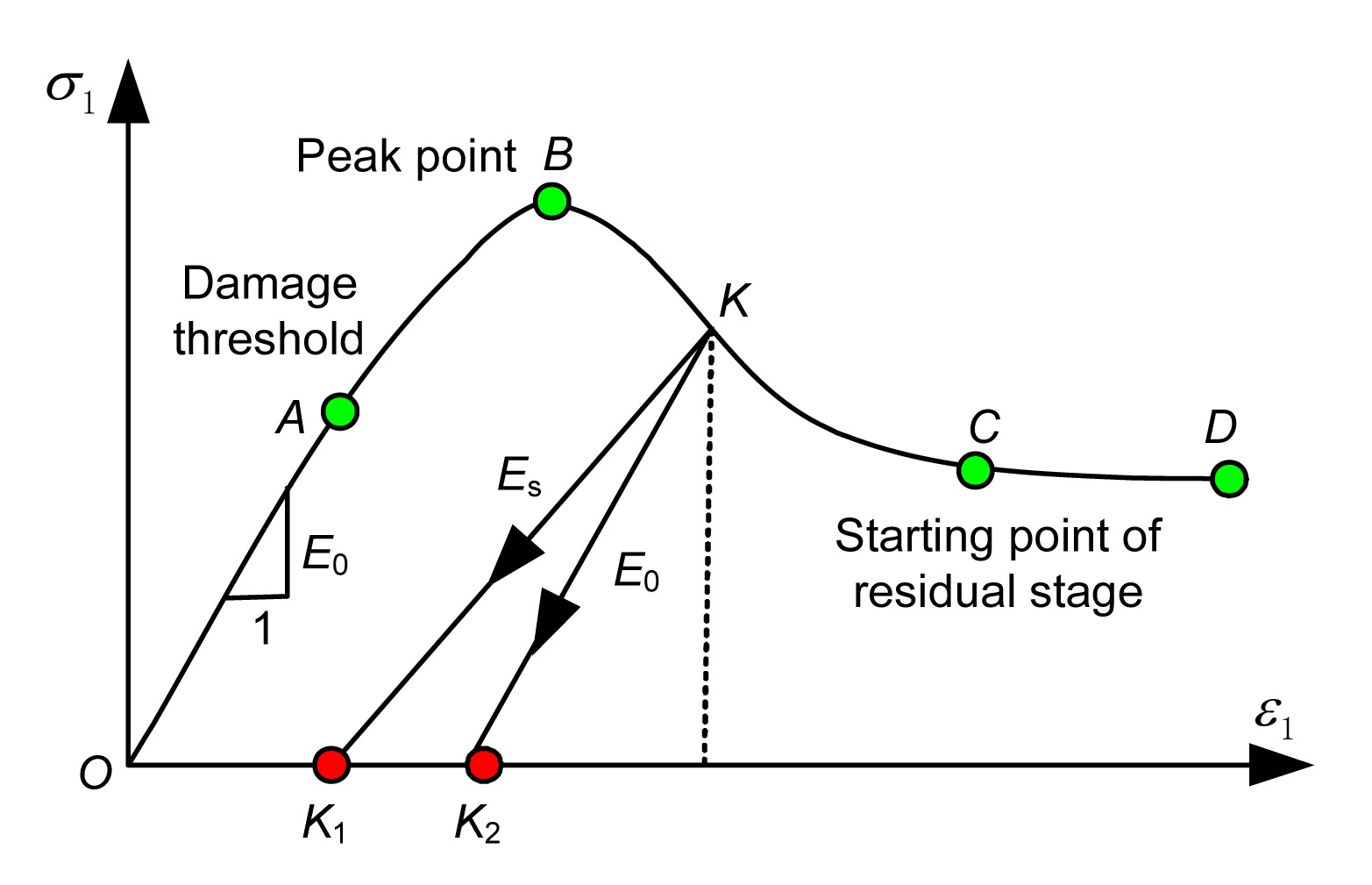Fig.2
Stiffness degradation of mudstone under tri-axial compression

The yield step before the peak point could be treated as an extension of the elastic range due to the relatively short feature of yield stage AB (Fig. 2). Therefore, the stress-strain curve of soft mudstone was simplified as a tri-linear model (Fig. 3) according to the plastic strain softening model proposed by Toshikazu (). Stage OB includes pressurization, closure, and the development of internal micro-cracks. In stage BC, the stiffness and strength of the rock sample gradually decrease due to the extension and penetration of cracks. Stage CD represents the residual stage. To consider the effect of confining pressure, an equivalent stress σ e=σ 1−2νσ 3 was defined as vertical coordinates without considering the change in Poisson’s ratio ν. In fact, the equivalent stress σ e is equal to the superposition of the axial stress σ 1 and a constant under a specified confining pressure, so the curve shapes of σ eε 1 and σ 1ε 1 are consistent with each other.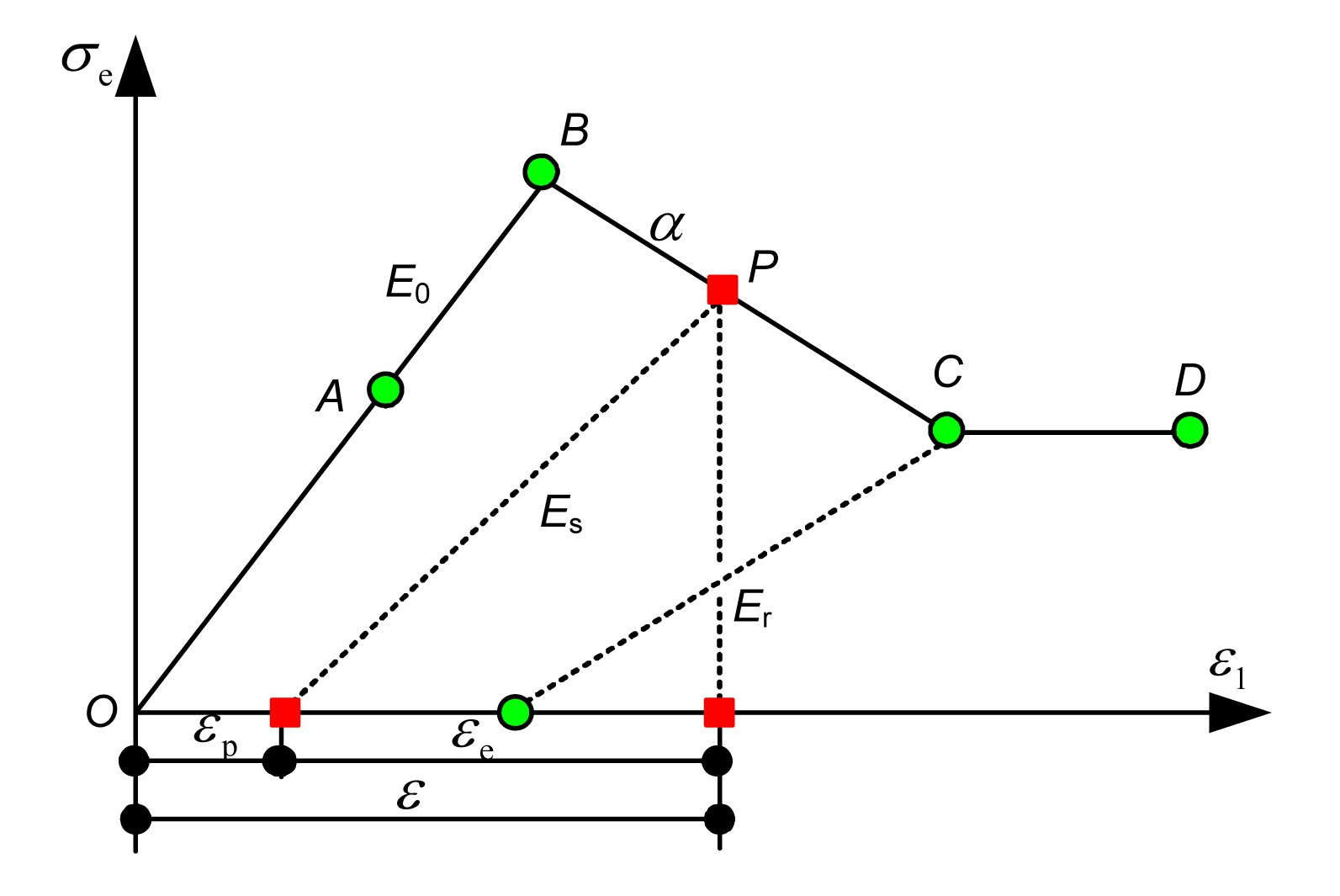Fig.3
Simplified tri-linear strain softening model of mudstone

Let the elastic modulus at the pre-peak stage and post-peak stage be E 0 and E s, respectively. Here, we introduce a softening modulus α, which denotes the softening degree at the post-peak stage. The relationship E c=E 0 should be satisfied without considering the stiffness attenuation at the yield stage, where E c represents the unloading modulus at the peak point. Through the introduction of a stiffness degradation coefficient ω with a scope of [0, 1], the unloading elastic modulus E s of point P at the post-peak stage can be defined as follows: , where E r represents the elastic modulus at the residual stage. Equalities E s=E 0 and E s=E r hold when ω is set as 0 and 1, respectively. Actually, E s is a function of ω under a specified confining pressure. Here, ε e and ε p denote the elastic strain and plastic strain of point P, respectively.

## 3.  Evolution model for strength parameters of soft rock

The equation for the post-peak stage can be expressed as , where σ 1c and σ 1 represent the axial stress at the peak point and point P, respectively. Suppose that ε 1c and ε 1 are the axial strains at the peak point and at point P, respectively. From Fig. 3, the total axial strain can be derived as , where ε 1e and ε 1p stand for the elastic strain and plastic strain, respectively. For conventional tri-axial compression, the elastic strain ε 1e of point P can be calculated as , thus the plastic strain should be .

Regardless of stiffness degradation, the elastic strain ε 1e in the post-peak stage can be calculated as ε 1e '=σ e/E 0. According to the relationship E s<E 0, it is evident that the plastic strain decreases with the stiffness attenuation caused by the damage mechanism due to ε 1e<ε 1e′. Actually, the deformation of soft rock is a coupled process of damage failure and a plastic mechanism. Suppose the stress state at the post-peak stage meets the Mohr-Coulomb yield criterion. Namely, all stress points are on yield surfaces Φ, which can be expressed as , where ξ=(1+sinφ)/(1−sinφ), C and φ denote the cohesion and friction angle, respectively, of the subsequent yield surface in the strain softening stage. It is clear that the cohesion C and friction angle φ are not constant due to the degradation of stiffness and strength during the post-peak stage. By selecting the equivalent plastic shear strain ε ps as a softening parameter, cohesion C and friction angle φ should be the function of the confining pressure σ 3 and ε ps. That is, the stress state on the subsequent yield surface should satisfy , Without considering the effect of intermediate principal stress σ 2, the equivalent plastic shear strain ε ps can be calculated as , where ε mp is the average plastic strain, which can be obtained from ε mp=(ε 1p+ε 3p)/3.

Let η be the dialtancy gradient according to the irrelative plastic flowing law. The lateral strain ε 1p can be calculated as . When η=1, no dialtancy effect exists. Only if η>1, will a dialtancy effect be produced in the softening stage. The dialtancy gradient η should be the function of the confining pressure, namely η=η(σ 3). According to the simultaneous solution of Eqs. (8) and (9), the equivalent plastic strain at the post-peak stage can be changed to , where . Based on Eqs. (3) and (4), the relationship between strength and plastic strain in the softening stage can be established as follows: . Let C 0 and φ 0 be the initial cohesion and friction angle respectively, of the rock sample. When the sample is loaded to peak point, only the initial yield is produced, and the initial yield surface should satisfy , where .

From Eq. (12), the axial stress σ 1c is calculated as . Thus, by jointly solving Eqs. (7), (10), (11), and (13), the evolution equations of strength parameters are determined as , , where

The internal friction angle reflects mainly the friction strength characteristics of soft rock, and its evolution law at the post-peak stage is dominated by the instantaneous stress state and damage mechanism. The attenuation law of cohesion is related to the plastic deformation behavior of soft rock, in addition to the stress level and damage state. Without considering the stiffness degradation, namely E s=E 0, the model factors are determined as A 1=1, A 2=0, and A 3 depends only on the softening modulus α. In this case, the friction angle at the softening stage will keep constant, and the cohesion will decrease in linear rule. This result is consistent with the conclusions obtained by Zhang et al. ().

## 4.  Engineering case

The mechanical parameters of soft mudstone collected from the Ili mining area were measured as follows, by a tri-axial compression experiment: initial cohesion C 0=4.5 MPa, initial friction angle φ 0=44°, and Poisson’s ratio ν=0.25. Calculation results for other model parameters are listed in Table 1.

#### Table 1

Test results of soft mudstone
 σ 3 (MPa) Model parameter α (GPa) E 0 (GPa) E s (GPa) ε 1c (%) σ 1c (MPa) η 0 2.68 1.51 0.31 0.43 9.33 1.83 1 2.60 1.55 0.84 0.61 11.20 1.56 2 2.54 1.68 0.92 0.64 12.40 1.34 3 2.50 1.84 1.12 0.68 13.50 1.21 4 2.38 2.21 1.88 0.88 21.80 1.08 5 2.26 3.18 2.54 1.16 29.90 1.02

Fig. 4 shows the calculation results of evolution laws of the friction angle under different confining pressures according to Eq. (14). Clearly, the degradation rate of the friction angle under uni-axial compression is significantly higher than that under tri-axial compression condition. Due to the characteristic of weak cementation, the friction angle of mudstone decreases to 20° when the confining pressure is set at 0 MPa. As the confining pressure increases, the attenuation rate decreases gradually and remains relatively constant when the confining pressure increases to 5 MPa. The percentages of the residual friction angle in relation to its initial value are 45.3%, 78.3%, 82.2%, 85.7%, 94.6%, and 98.0%, respectively, with confining pressure increasing from 0 MPa to 5 MPa.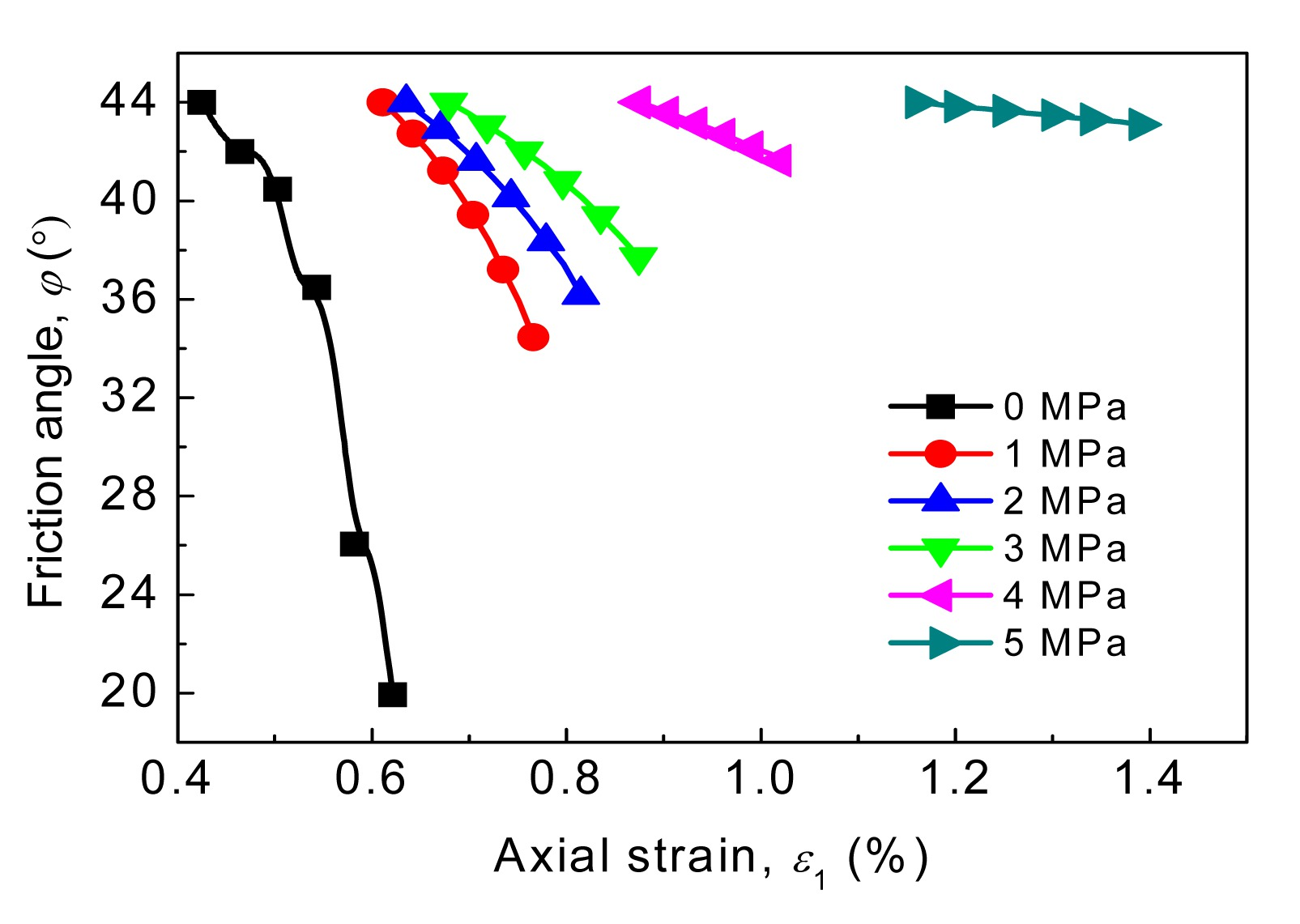Fig.4
Evolution laws of the friction angle under different confining pressures

The attenuation laws of cohesion at the post-peak stage under different confining pressures according to Eq. (15) are shown in Fig. 5. As for the friction angle, the cohesion decreases rapidly under uni-axial compression. With increasing confining pressures, the attenuation rate seems to head downwards. Even if the confining pressure is increased to 5 MPa, cohesion of soft mudstone exhibits an obvious attenuation tendency. The percentages of residual cohesion in relation to its initial value are 45.3%, 72.2%, 74.2%, 75.9%, 84.0%, and 87.1%, respectively, when the confining pressure is increased from 0 MPa to 5 MPa.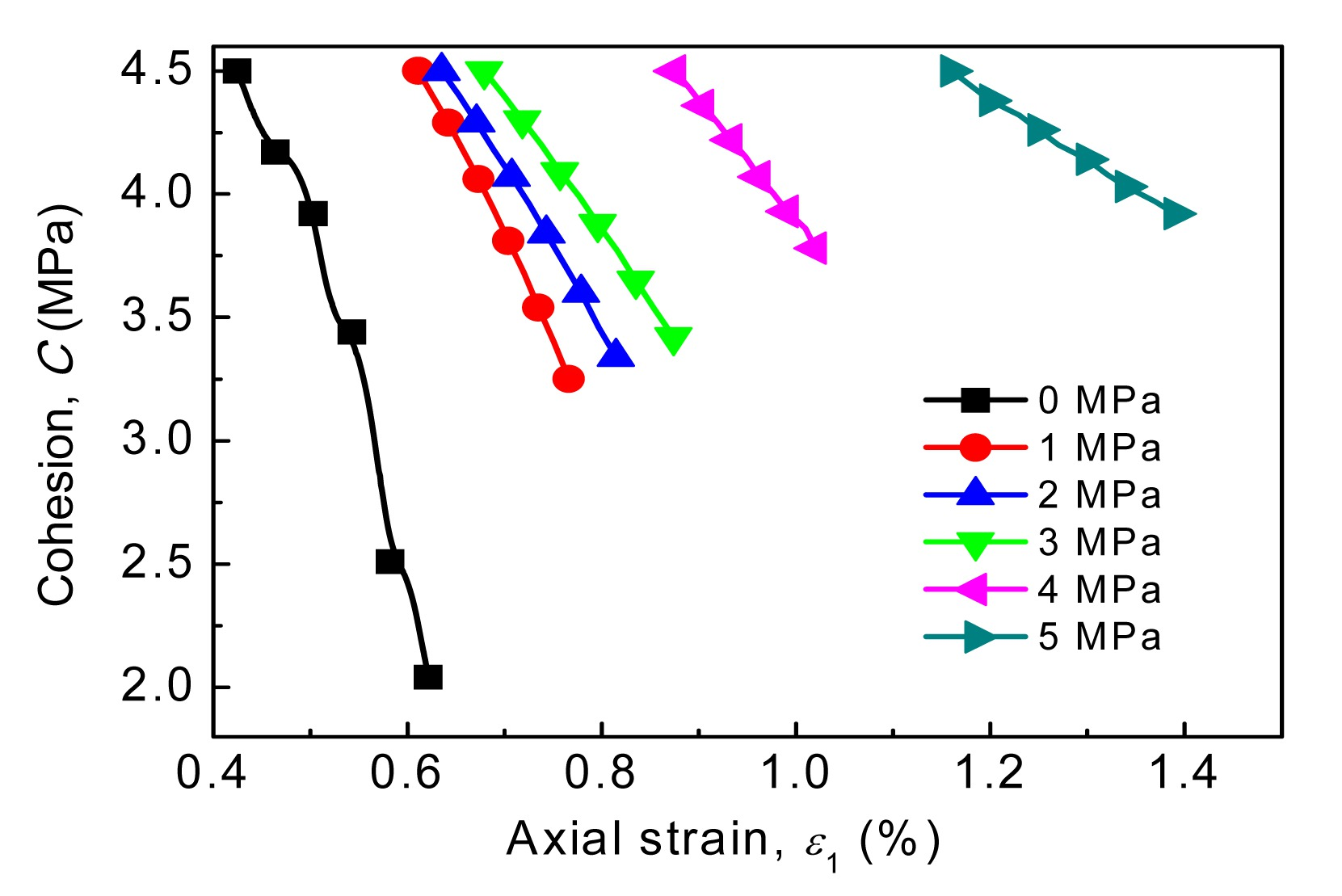Fig.5
Evolution laws of cohesion under different confining pressures

Fig. 6 shows a comparison of residual strength parameters of soft mudstone under different confining pressures. The residual cohesion and friction angle improve significantly with increasing confining pressure. Their growth rates under high confining pressure tend to be stable. The residual friction angle approaches its initial value before damage occurs.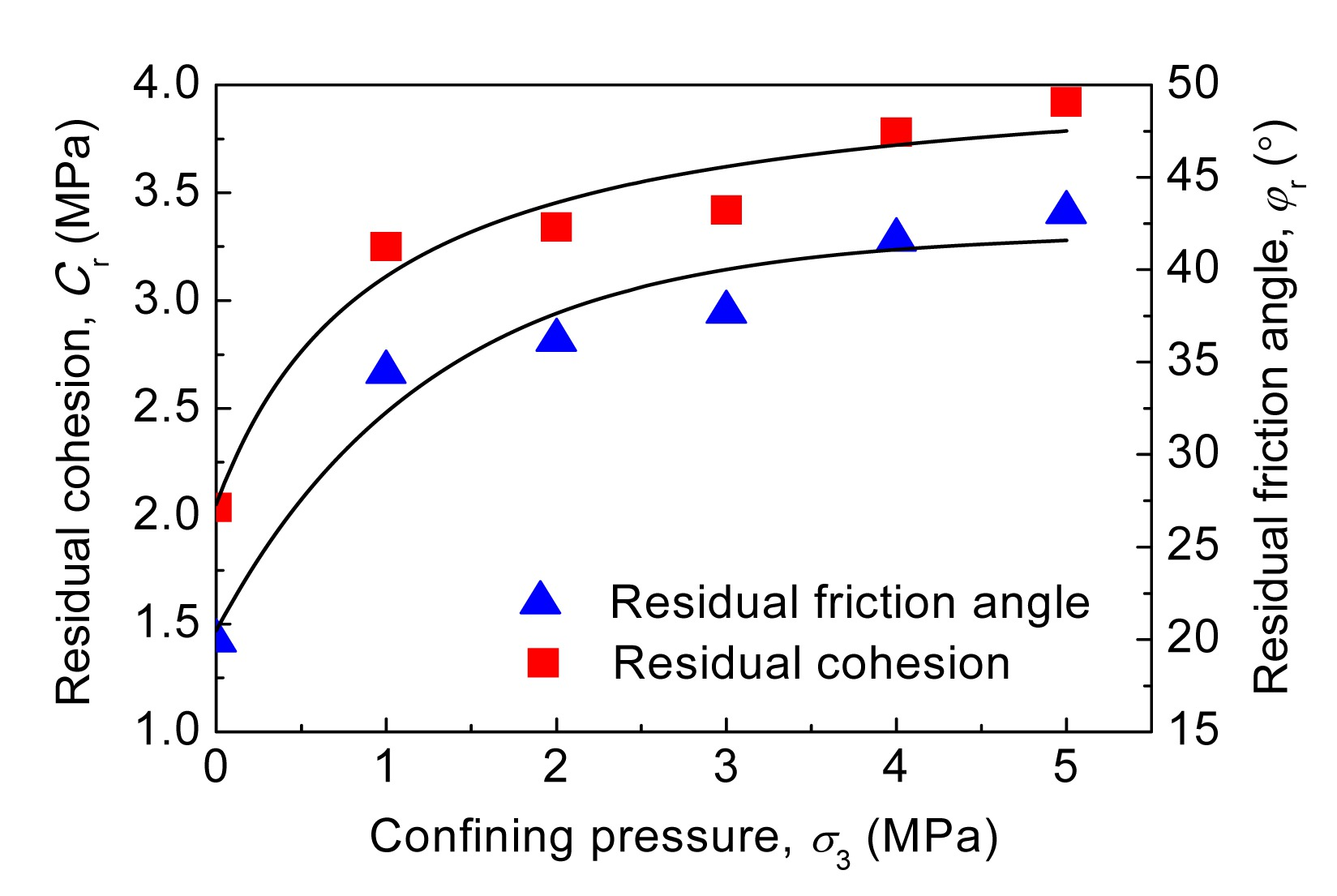Fig.6
Residual strength parameters under different confining pressures

The relationship between the residual friction angle φ r and the confining pressure σ 3 is established by data regression, as follows: . By adopting the same practice, the regression equation for residual cohesion C r is calculated from .

For further analysis of the corresponding relationships between degradation stiffness and strength parameters with confining pressure and equivalent plastic strain, a contour plot for the evolution laws of mechanical parameters at the post-peak stage was drawn (Fig. 7). The results were acquired by 3D data fitting in MATLAB.Fig.7
Evolution contours of mechanical parameters of soft mudstone under different confining pressures
Contour plot for stiffness degradation (a), the evolution of cohesion (b), and the evolution of the friction angle (c)

The change tendency of the evolution contour plot of elastic modulus at the post-peak stage is shown in Fig. 7a. The contour value alters sharply under low confining pressure (≤3 MPa), which indicates that the stiffness has severely decreased. For instance, the percentage of residual stiffness in relation to its initial value is only 20%, 54%, 55%, and 60% when the confining pressure is set at 0 MPa, 1 MPa, 2 MPa, and 3 MPa, respectively. However, if σ 3 is increased to 4 MPa and 5 MPa, it increases to 75% and 78%, respectively. The change tendency of the evolution contour plot reveals the significant effect of confining pressure on stiffness degradation and plastic deformation.

Figs. 7b and 7c show the evolution contour plots of the cohesion and friction angle, respectively, with equivalent plastic strain under different confining pressures. Under the action of low confining pressure, the strength parameters decrease dramatically with equivalent plastic strain at the post-peak stage. Results of equivalent plastic strain show significant differences under different confining pressures. When σ 3>3 MPa, the contour plot changes smoothly, which represents a low attenuation rate of strength parameters.

## 5.  Model verification

To verify the advantages of the model proposed in this work, an indoor tri-axial compression experiment and corresponding numerical simulation were carried out and the results were compared. The strain softening model embedded in FLAC3D was established under the assumption that the strength parameters decrease linearly with the equivalent plastic shear strain, without considering the stiffness degradation at the post-peak stage. To address this deficiency, based on Fish programming language, the embedded model in FLAC3D was modified according to the evolution model determined by Eqs. (14) and (15). The mechanical parameters for the numerical simulation are listed in Table 2.

#### Table 2

Physical parameters of mudstone
 Sample E (MPa) ν C (MPa) φ (°) σ t (MPa) Mudstone 2100 0.25 4.5 44 1.11

• Note: σ t represents the tensile strength of mudstone

•  Fig. 8 shows the numerical simulation model for the mudstone sample. The loading mode is controlled by displacement with an axial velocity of v=1×10−8 m/s, and the confining pressure was set at 0 MPa, 1 MPa, 3 MPa, 4 MPa, and 5 MPa.Fig.8
Numerical calculation model of mudstone under tri-axial compression

A comparison of the results from the indoor experiment and the numerical simulation is shown in Fig. 9. The simulation results were in good agreement with the experimental results, and the two showed the same change tendency under different confining pressures. The proposed model can accurately describe the residual stage under low confining pressure. However, the simulation results were slightly higher than the experimental results under high confining pressure (3 MPa, 4 MPa, and 5 MPa). On the whole, the peak strength of the simulation results was a little higher than that of the experimental results. This is due to the complexity of damage evolution caused by the development, extension and perforation of micro-cracks in the mudstone sample which cannot be considered in the simulation model. The simulation results showed a small fluctuation near the peak point when σ 3 was set at 5 MPa. This may result from inhomogeneous deformation caused by strain localization.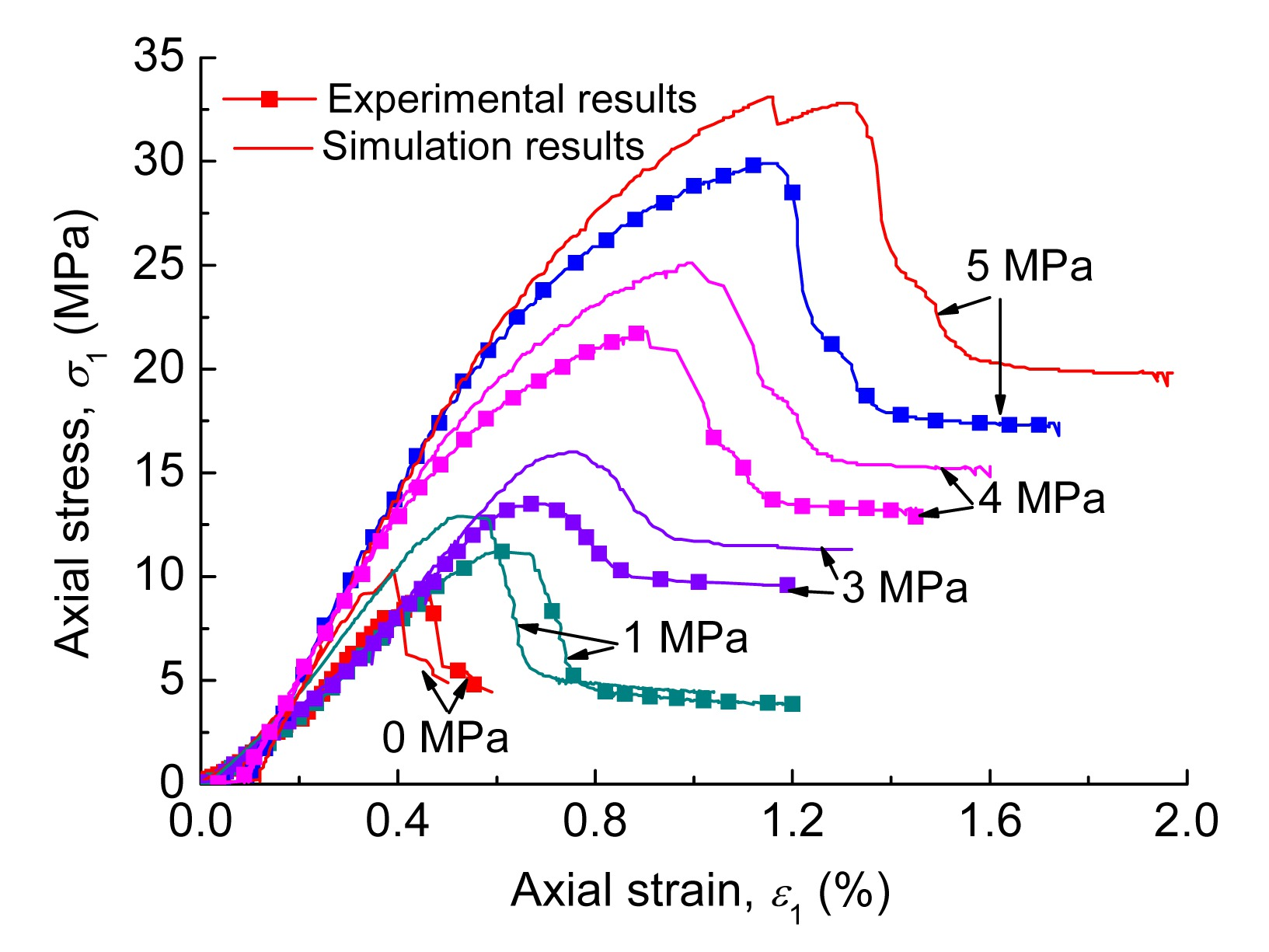Fig.9
Comparison of experimental and numerical calculation results for mudstone

## 6.  Conclusions

From the results of tri-axial experiments and our evolution model for strength parameters considering stiffness degradation, we draw the following conclusions:

1. The damage threshold of soft mudstone under compression is at the yield point. However, the yield step before the peak point can be treated as an extension of the elastic range due to the relatively short feature and low damage level.

2. Stiffness degradation behavior has a significant influence on the evolution laws of strength parameters. The evolution rule of the friction angle is dominated mainly by the instantaneous stress state and the damage mechanism. The attenuation law of cohesion is related to the plastic behavior in addition to the stress level and damage mechanism.

3. Strength parameters of mudstone show fast attenuation under low confining pressure, and the rate slows down with increasing confining pressure. The friction angle tends towards its initial value under high confining pressure. All the residual strength parameters are enhanced under high confining pressure.

4. Our evolution model considering stiffness degradation in a mudstone sample can accurately demonstrate the attenuation law of strength parameters, thereby providing a theoretical basis for the further study of stability mechanisms of roadways excavated in soft rock strata.

* Project supported by the National Natural Science Foundation of China (No. 51174128), and the Specialized Research Fund for the Doctoral Program of Higher Education of China (No. 20123718110007)

## ReferencesCao, W.G., Li, X., Zhao, H., 2007. Damage constitutive model for strain-softening rock based on normal distribution and its parameter determination. Journal of Central South University of Technology, 14(5):719-724.Chen, G.Q., Feng, X.T., Jiang, Q., 2010. Study of dynamic early warning method of surrounding rock deformation monitoring for large underground powerhouse considering rock degradation. Rock and Soil Mechanics, (in Chinese),31(9):3012-3018.Fang, Z., Harrison, J.P., 2001. A mechanical degradation index for rock. International Journal of Rock Mechanics and Mining Sciences, 38(8):1193-1199.Flora, A., Lirer, S., 2013. Small strain shear modulus of undisturbed gravelly soils during undrained cyclic triaxial tests. Geotechnical and Geological Engineering, 31(4):1107-1122.Han, J.X., Li, S.C., Yang, W.M., 2013. Study of post-peak stress-strain relationship of rock material based on evolution of strength parameters. Rock and Soil Mechanics, (in Chinese),34(2):342-346.Hoek, E., Brown, E.T., 1997. Practical estimates of rock mass strength. International Journal of Rock Mechanics and Mining Sciences, 34(8):1165-1187.Isik, N.S., Doyuran, V., Ulusay, R., 2008. Assessment of deformation modulus of weak rock masses from pressuremeter tests and seismic surveys. Bulletin of Engineering Geology and the Environment, 67(3):293-304.Joseph, T.G., 2000.  Estimation of the Post-failure Stiffness of Rock. PhD Thesis, University of Alberta,Alberta, Canada :Joseph, T.G., Barron, K., 2003. The post-failure characteristics of rock. CIM Bulletin, 96(1070):66-76.Lei, T., Zhou, K.P., Hu, J.H., 2013. Mechanics parameters deterioration laws of unloading rock by meso-damage mechanics. Journal of Central South University (Science and Technology), (in Chinese),44(1):275-281.Li, Y.J., Zhang, D.L., Liu, B.G., 2011. Development and verification of strain-softening model considering deformation modulus degradation in FLAC3DRock and Soil Mechanics, (in Chinese),32(S2):647-659.Lu, Y.L., Wang, L.G., Yang, F., 2010. Post-peak strain softening mechanical properties of weak rock. Chinese Journal of Rock Mechanics and Engineering, (in Chinese),29(3):640-648.Martin, C.D., 1997. Seventeenth Canadian geotechnical colloquium: the effect of cohesion loss and stress path on brittle rock strength. Canadian Geotechnical Journal, 34(5):698-725.Martin, C.D., Chandler, N., 1994. The progressive fracture of Lac du Bonnet granite. International Journal of Rock Mechanics and Mining Sciences & Geomechanics Abstracts, 31(6):643-659.Niu, S.J., Jing, H.W., Yang, X.X., 2012. Experimental study of strength degradation law of surrounding rock in fractured zone of deep roadway. Chinese Journal of Rock Mechanics and Engineering, (in Chinese),31(8):1587-1596.Palchik, V., 2011. On the ratios between elastic modulus and uniaxial compressive strength of heterogeneous carbonate rocks. Rock Mechanics and Rock Engineering, 44(1):121-128.Toshikazu, K., 1981. An analysis of excavation in strain-softening rock. Proceedings of the Japan Society of Civil Engineers, :107-117.Wang, S.L., Wang, W., Wu, Z.J., 2010. Study of relationship between evolution of post-peak strength parameters and stress-strain curves of geo-materials. Chinese Journal of Rock Mechanics and Engineering, (in Chinese),29(8):1524-1529.Wang, X.B., Pan, Y.S., Dai, S.H., 2009. Stability analysis of shear-band and surrounding-rock system considering stiffness deterioration. Journal of Disaster Prevention and Mitigation Engineering, (in Chinese),29(2):147-153.Yu, H.J., Zhang, C.H., Wang, L.G., 2012. Post-peak strain softening model of rock based on degradation angle. Journal of China Coal Society, 37(3):402-406.Zdenek, P., Bazant, F., 1984. Continuum theory for strain-softening. Journal of Engineering Mechanics, 110(12):1666-1692.Zhang, F., Sheng, Q., Zhu, Z.Q., 2008. Study of post-peak mechanical behavior and strain-softening model of Three Gorges granite. Chinese Journal of Rock Mechanics and Engineering, (in Chinese),27(S1):2651-2655.Zhang, K., Zhou, H., Shao, J.F., 2013. An experimental investigation and an elastoplastic constitutive model for a porous rock. Rock Mechanics and Rock Engineering, 46(6):1499-1511.